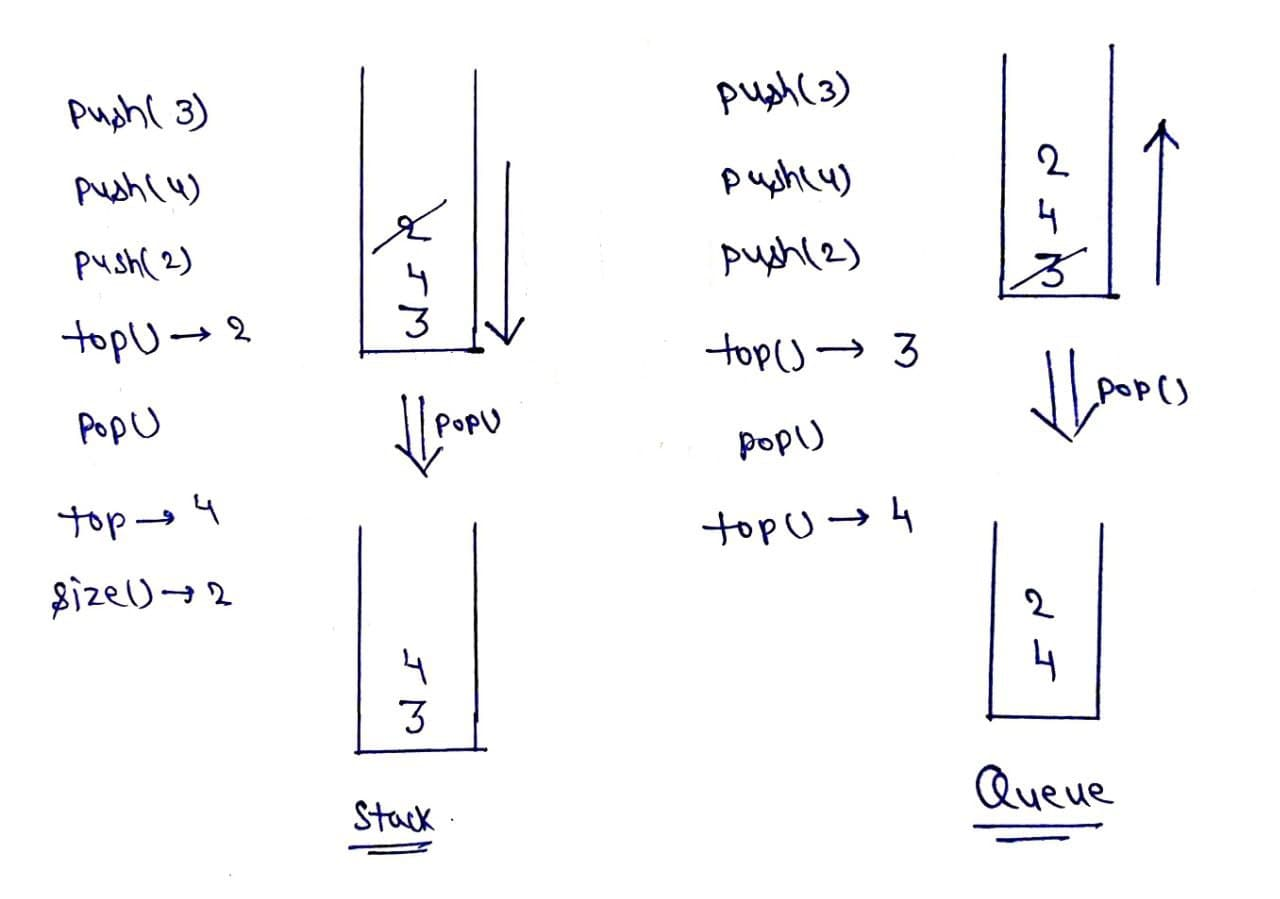# Implement Stack using single Queue

Problem Statement: Implement a Stack using a single Queue.

Note: Stack is a data structure that follows the Last In First Out (LIFO) rule.

Note: Queue is a data structure that follows the First In First Out (FIFO) rule.

Example:

```Explanation:
push(): Insert the element in the stack.
pop(): Remove and return the topmost element of the stack.
top(): Return the topmost element of the stack
size(): Return the size of the stack
```

Solution:

Disclaimer: Don’t jump directly to the solution, try it out yourself first.

Intuition: As we know stack follows last in first out, which means we get the most recently inserted element whenever we remove an element from the stack. But queue follows first in first out, it means we get that element which we inserted in the starting at each deletion, it means if we want to use the queue like a stack we have to arrange elements in the queue such that we get the most recent element at each deletion.

Approach:

• Take a single queue.
• push(x): Push the element in the queue.
• Use a for loop of size()-1, remove element from queue and again push back to the queue, hence the most recent element becomes the most former element and vice versa.
• pop(): remove the element from the queue.
• top(): show the element at the top of the queue.
• size(): size of the current queue.

Repeat step3 at every insertion of the element.Code:

## C++ Code

``````#include<bits/stdc++.h>

using namespace std;

class Stack {
queue < int > q;
public:
void Push(int x) {
int s = q.size();
q.push(x);
for (int i = 0; i < s; i++) {

q.push(q.front());
q.pop();
}
}
int Pop() {
int n = q.front();
q.pop();
return n;
}
int Top() {
return q.front();
}
int Size() {
return q.size();
}
};

int main() {
Stack s;
s.Push(3);
s.Push(2);
s.Push(4);
s.Push(1);
cout << "Top of the stack: " << s.Top() << endl;
cout << "Size of the stack before removing element: " << s.Size() << endl;
cout << "The deleted element is: " << s.Pop() << endl;
cout << "Top of the stack after removing element: " << s.Top() << endl;
cout << "Size of the stack after removing element: " << s.Size();

}``````

Output:

Top of the stack: 1
Size of the stack before removing element: 4
The deleted element is: 1
Top of the stack after removing element: 4
Size of the stack after removing element: 3

Time Complexity: O(N)

Space Complexity: O(N)

## Java Code

``````import java.util.*;

public class tuf {

public static void main(String[] args) {
stack s = new stack();
s.push(3);
s.push(2);
s.push(4);
s.push(1);
System.out.println("Top of the stack: " + s.top());
System.out.println("Size of the stack before removing element: " + s.size());
System.out.println("The deleted element is: " + s.pop());
System.out.println("Top of the stack after removing element: " + s.top());
System.out.println("Size of the stack after removing element: " + s.size());
}

}
class stack {
Queue < Integer > q = new LinkedList < > ();
void push(int x) {
for (int i = 0; i < q.size() - 1; i++) {
}
}
int pop() {
return q.remove();
}
int top() {
return q.peek();
}
int size() {
return q.size();
}
}``````

Output:

Top of the stack: 1
Size of the stack before removing element: 4
The deleted element is: 1
Top of the stack after removing element: 4
Size of the stack after removing element: 3

Time Complexity: O(N)

Space Complexity: O(N)

## Python Code

``````from queue import Queue

class Stack:
def __init__(self):
self.q = Queue()

def push(self, x):
s = self.q.qsize()
self.q.put(x)
for i in range(s):
self.q.put(self.q.get())

def pop(self):
n = self.q.get()
return n

def top(self):
return self.q.queue

def size(self):
return self.q.qsize()

if __name__ == "__main__":
s = Stack()
s.push(3)
s.push(2)
s.push(4)
s.push(1)
print("Top of the stack: ", s.top())
print("Size of the stack before removing element: ", s.size())
print("The deleted element is: ", s.pop())
print("Top of the stack after removing element: ", s.top())
print("Size of the stack after removing element: ", s.size())``````

Output:

Top of the stack: 1
Size of the stack before removing element: 4
The deleted element is: 1
Top of the stack after removing element: 4
Size of the stack after removing element: 3

Time Complexity: O(N)

Space Complexity: O(N)